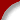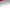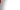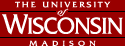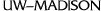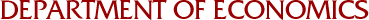# Econ 410- Introductory Econometrics

Correction to the syllabus: the link to the introduction to STATA doesn't work any longer. Instead, you may consult the following address for STATA study: http://en.wikibooks.org/wiki/stata/introduction.

Lecture 1|| 2 || 3 || 4 || 5 || 6 || 7 || 8 || 9 || 10 || 11 || 12 || 13 || 14 || 15 || 16 || 17 || 18 || 19 || 20 || 21 || 22 ||

Problem set 1 sol || 2 sol || 3 sol || 4 sol || 5 sol || 6 sol ||

Midterm 2. Midterm with Solution || Grade Analysis

Final. Review Material || Practice Exam

Lecture 1: Introductory Lecture

Lecture 2: Review of Statistics

Lecture 3: Simple Regression I

Lecture 4: Simple Regression II

Readings: Chapter 2 of Wooldridge (Ch2.5)

Lecture 5: Multiple Regression I

Lecture 6: Multiple Regression II

Lecture 7: Inference in Regression Models

Lecture 8: Inference in Regression Models (Continue)

Lecture 9: Inference in Regression Models (Continue)

Lecture 10: Review Lecture

Lecture 12: Asymptotic Properties of OLS Estimators - Continue

Lecture 13: Dummy Variables

Lecture 14: Dummy Variables - Continue, Exercise

Lecture 15: Heteroskedasticity , Exercise

Readings: Chapter 8 of Wooldridge, 8.1-2

Lecture 16: Heteroskedasticity - Continue, Exercise

Readings: Chapter 8 of Wooldridge, 8.3-4

Lecture 17: Endogeneity, Exercise

Readings: Chapter 9 of Wooldridge, 9.1-2

Readings: Chapters 9.4 and 15.1-3 of Wooldridge

Lecture 19: Pooled Cross Section and Panel Data

Lecture 20: Time Series - Introduction Exercises

Lecture 21: Time Series - Large Sample Theory Exercises